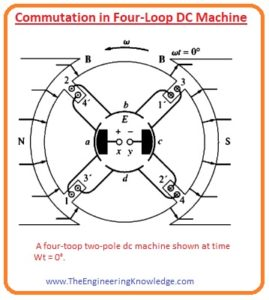Hello, fellows, I hope all of you are having fun in your life. In today’s tutorial, we will discuss the Commutation in Four-Loop DC Machine. The voltage generated at output terminals dc machines are pure AC voltage, to converts these voltages into dc voltage commutators are introduced at the terminals that convert in DC voltages this process of AC to DC is known as the commutation. To a simple understanding of voltage induced dc machines you read a simple loop in field it will clear your concepts how ac voltage induced in dc machines.

In today’s post, we will have a detailed look at how ac voltage is converted in the dc by using commutators and some other related parameters. So let’s get started with Commutation in Four-Loop DC Machine.

#### Commutation in Four Loop DC Machine

• For simple study of commutation in DC machines we take a dc machine that has 4 windings loop, and 2-poles as it is shown in a given figure.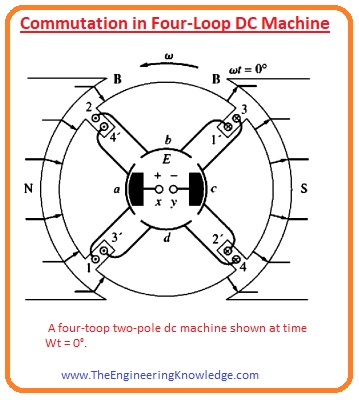• These windings loops are wounded at the rotor in the slots the rotor is constructed by the steel.
• The poles at the stator are arched shaped to provides identical flux in the machine and have a uniform air gap.
• In the above given figure, you can see that the 4 windings loops are arranged at the rotor in a special technique.
• The primed end of every winding loop is the inner end of the wire and the un-primed end of every windings loop is the outer end of the wire.
• The linking of windings with the commutator is shown in a given figure.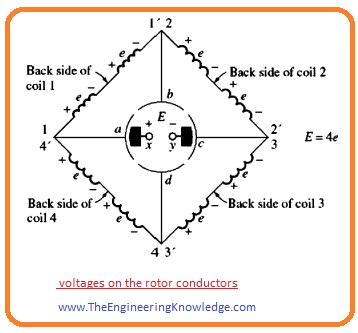• You can see that the first windings loop is linked with the commutator segments a and b and the second windings loop is joined with the commutator points b and c.
• In given below figure you can see that these windings loops (1, 2, 3’) and 4’are under the effect of the south pole. The value of voltages at these windings loop is given as.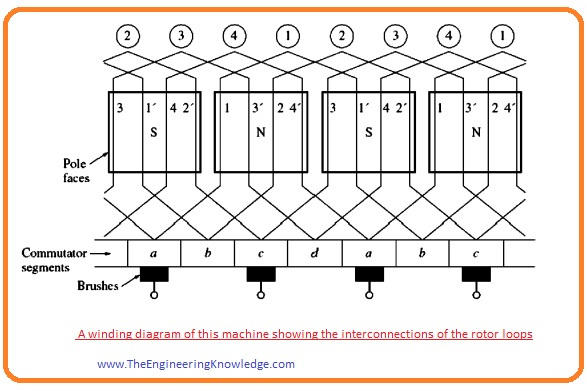eind = (v x B) • I

eind = VBl positive out of page

• The other ends of windings (1’ 2′, 3, 4) that are under the effect of the north pole have a value of voltage as.

eind = (v x B) • I

eind = VBl positive into page

• The complete outcome represented by this given below figure ) every conductor recognized as one side of the winding loop.• If the voltage produced at every conductor of winding coil is (vBl) then the net value of the voltage at the output terminals of the dc machine is given as.
• E= 4e, at wt=0
• You can see that there are 2 parallel paths for current in a machine. The availability of 2 or more parallel paths is the general outcome of the commutation process.
• Here one question arises.
• What is the effect of rotor’s continues rotation of output voltages E?
• The given figure shows the machine at the time (wt=450).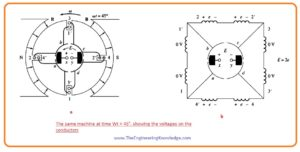• At this time the first and third winding loop is rotating in the gap among the poles, consequently, the value of voltage is 0.
• You can see that during this time the brushes are connected with the commutator segments ab and cd.
• It occurs when the voltage at windings is zero volts, so it creates no problem (connection of commutators segments).
• The windings loops second and fourth are in effect of poles si the output voltage will be.

E=2e, wt=00

• Let’s suppose that the rotor moves for another 450. This condition is shown in this below given picture.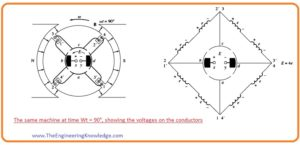• In this case, the (1′,2,3,4′) ends of windings are an effect of N pole and (1, 2’, 3’, 4) are facing the S pole.
• In this situation the voltage produced for loop’s ends below the N pole is out of page and for other end is into a page.
• Now the 4 current carrying ends in every parallel path thus the output voltage will be.
• E =4e, wt =900
• If we compare these 2 given figures (A) and (B) voltage on first and third windings loop have inverted among two circuits, their joining points also altered, but the voltage production is still similar as earlier.
• It is the very important parameter of each commutation arrangement, when the voltage converses in a windings loop, the connections of the loop are also changed, but the net voltage are still producing in the original direction.
• The output voltage of the machine is shown in this given figure.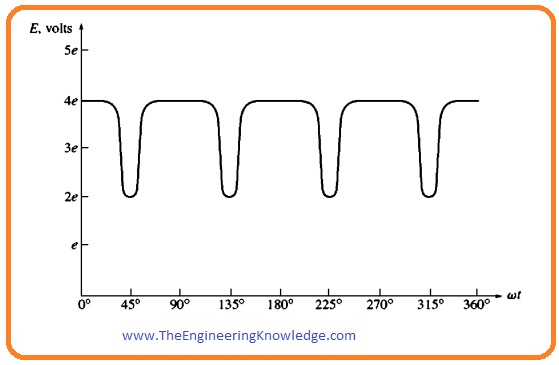#### Summary

• Commutation is the procedure of swapping the loop connections on the rotor of a direct current machine like the voltage in the loop shifts polarity, to provide constant dc voltage at output terminals.

That is a complete post on Commutation in Four Loop DC Machine if you have any questions about this tutorial ask in comments. See you in the next tutorial.

1. 2020 says:

Hi, I do believe this is a great website. I stumbledupon it 😉 I’m
going to come back yet again since i have book marked it.
Money and freedom is the greatest way to change, may you be rich and continue to help others.

2. Martin Dooney says:

I am struggling to achieve commutation in a 4 pole, 2 brush DC motor.
Field V are 100/200 Arm V are 180
A1 & A2 should just dictate the polarity of the armature.

There are 4 F leads, which I have connected as F1&F3, F2&F4 and also F1, F2&F3, F4.

When we apply 100V to whichever connection as mentioned above on the field system and then try to excite the armature, it’s as if something internally is fighting as the armature tries to spin, but skips and then repeats without ever fully exciting, regardless of the voltage that is applied.

Any suggestions?